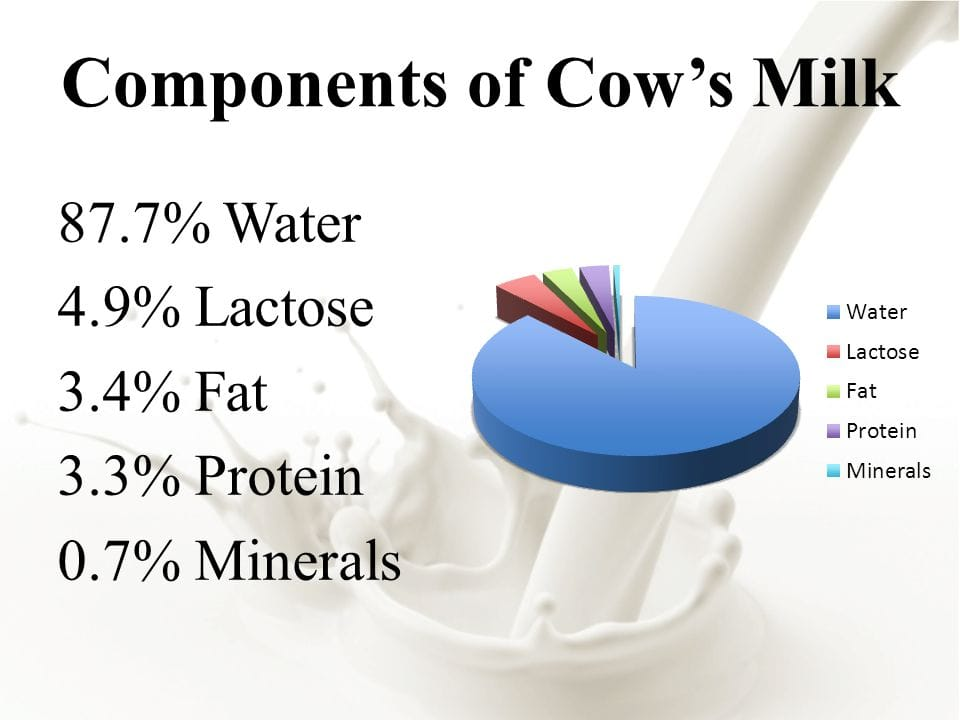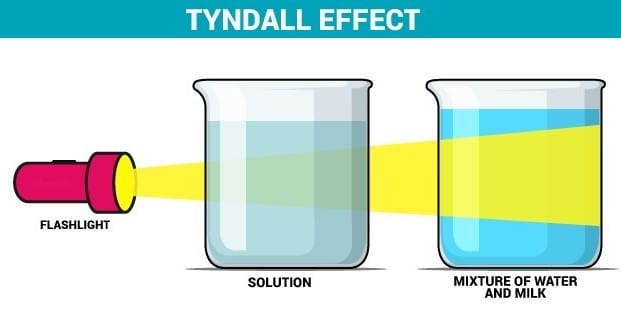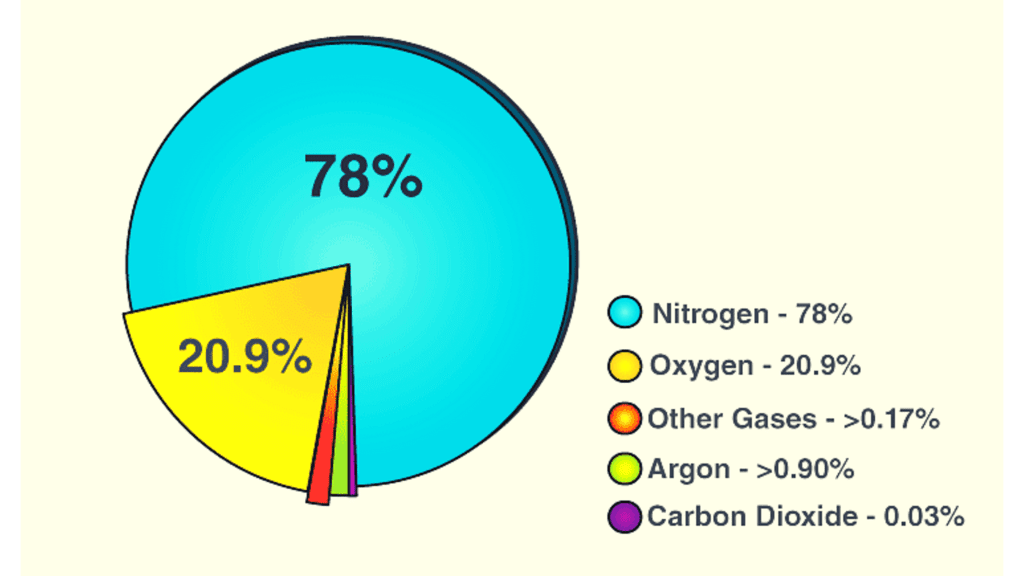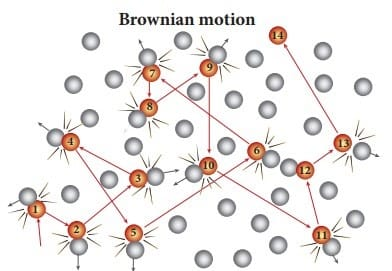Is Matter Around Us Pure: Assertion & Reason Type Questions

# Is Matter Around Us Pure: Assertion & Reason Type Questions - Science Class 9

Direction: In the following questions, a statement of Assertion (A) is followed by a statement of Reason (R). Mark the correct choice.

Question 1:
Assertion: Oxygen is an element.
Reason: It cannot be decomposed by a physical or a chemical process.

(a) Both (A) and (R) are true, and (R) is the correct explanation of (A).
(b) Both (A) and (R) are true, but (R) is not the correct explanation of (A).
(c) (A) is true, but (R) is false.
(d) (A) is false, but (R) is true.

• Both assertion and reason statements are correct, and the reason is the correct explanation of assertion.
•  A substance that cannot be decomposed by a physical or a chemical process is known as an element.

Question 2:
Assertion: A solution is a homogeneous mixture of two or more chemically non-reacting substances.
Reason: Solution is prepared by the combination of two same phases of matter.

(a) Both (A) and (R) are true, and (R) is the correct explanation of (A).
(b) Both (A) and (R) are true, but (R) is not the correct explanation of (A).
(c) (A) is true, but (R) is false.
(d) (A) is false, but (R) is true.

• A solution is a homogeneous mixture of two or more chemically non-reacting substances.
• Solution is prepared by the combination of two same or different phases of matter.
• On the basis of phases of matter solution can be homogeneous and heterogeneous solution.
• Homogeneous means that the components of the mixture form a single phase.
• Heterogeneous means that the components of the mixture are of different phase
• Hence Option C is correct. Assertion is true but reason is false.

You can cover in detail the concepts of Mixtures and Solutions here.

Question 3:
Assertion: Hot water is used for the separation of benzoic acid from naphthalene.
Reason: Whenever a crystal is formed it tends to leave out the impurities.
(a) Both (A) and (R) are true, and (R) is the correct explanation of (A).
(b) Both (A) and (R) are true, but (R) is not the correct explanation of (A).
(c) (A) is true, but (R) is false.
(d) (A) is false, but (R) is true.

Benzoic acid dissolves in hot water but naphthalene does not therefore, hot water is used for separation of benzoic acid from naphthalene.

Question 4:
Assertion: Milk is a pure substance.
Reason: Milk can be separated by physical process into its components. It has components like water, fat, proteins etc.
(a) Both (A) and (R) are true, and (R) is the correct explanation of (A).
(b) Both (A) and (R) are true, but (R) is not the correct explanation of (A).
(c) (A) is true, but (R) is false.
(d) (A) is false, but (R) is true.

Milk is not a pure substance, it can be separated by physical process into its components. It has components like water, fat, proteins etQuestion 5:
Assertion: A mixture of glucose and m-dinitrobenzene can be separated by shaking it with ether.
Reason: Glucose is soluble in water.
(a) Both (A) and (R) are true, and (R) is the correct explanation of (A).
(b) Both (A) and (R) are true, but (R) is not the correct explanation of (A).
(c) (A) is true, but (R) is false.
(d) (A) is false, but (R) is true.

Since ether is not a polar solvent and does not form hydrogen bonds in solution so glucose is insoluble in ether but soluble in polar solvent like water.

Question 6:
Assertion: A solution can scatter a beam of light passing through it.
Reason: The particles of the solution are smaller than 1 nm in diameter.

(a) Both (A) and (R) are true, and (R) is the correct explanation of (A).
(b) Both (A) and (R) are true, but (R) is not the correct explanation of (A).
(c) (A) is true, but (R) is false.
(d) (A) is false, but (R) is true.

• A solution does not scatter a beam of light passing through it as the size of the particles of solution is smaller.
• According to the Tyndall effect light beams scatter owing to the existence of large colloidal particles in the solution that separates the light
• When a light goes through the genuine solution, the direction of light is invisible because the particles in the proper solution are small than 1 nm and hence cannot scatter the light, preventing the Tyndall effect from occurring.Question 7:
Assertion: A mixture of camphor and ammonium chloride cannot be separated by sublimation.
Reason: Camphor on heating sublimes, ammonium chloride does not.
(a) Both (A) and (R) are true, and (R) is the correct explanation of (A).
(b) Both (A) and (R) are true, but (R) is not the correct explanation of (A).
(c) (A) is true, but (R) is false.
(d) (A) is false, but (R) is true.

• The mixture of camphor and ammonium chloride cannot be separated by sublimation method since both are volatile substances. Thus, the mixture is dissolved in water.
• Ammonium chloride is soluble in water while camphor is not thus the water containing ammonium chloride is decanted in another beaker. Thus camphor is obtained and now the solution is evaporated to separate ammonium chloride.
•  The solution containing ammonium chloride is heated and boiled off and the ammonium chloride is left behind.

Question 8:
Assertion: Air is a mixture.
Reason: Air can be separated into its constituents like oxygen, nitrogen etc. by the physical process of fractional distillation.
(a) Both (A) and (R) are true, and (R) is the correct explanation of (A).
(b) Both (A) and (R) are true, but (R) is not the correct explanation of (A).
(c) (A) is true, but (R) is false.
(d) (A) is false, but (R) is true.

Air is a mixture, because it can be separated into its constituents like oxygen, nitrogen etc. by the physical process of fractional distillation. Also, air shows the properties of all the gases present in it.Composition of air

Question 9:
Assertion: Chloroform and benzene form a pair of miscible liquids and they are separated by fractional distillation.
Reason: The boiling point of benzene is less than that of chloroform.

(a) Both (A) and (R) are true, and (R) is the correct explanation of (A).
(b) Both (A) and (R) are true, but (R) is not the correct explanation of (A).
(c) (A) is true, but (R) is false.
(d) (A) is false, but (R) is true.

Chloroform (b.pt. 60ºC) and benzene (b.pt. 80º) can be separated by fractional distillation since fractional distillation is used for the separation of two or more liquids with difference in their boiling points of less than 25ºC. But the boiling point of benzene is more than chloroform.

Question 10:
Assertion: Alloys are a homogenous mixture of metals.
Reason: Alloys cannot be separated into their components by physical methods.

(a) Both (A) and (R) are true, and (R) is the correct explanation of (A).
(b) Both (A) and (R) are true, but (R) is not the correct explanation of (A).
(c) (A) is true, but (R) is false.
(d) (A) is false, but (R) is true.

• An alloy is a homogenous mixture of two or more metals or non-metals or nonmetals mixed in a molten state. It can either be a solid solution of the elements of a single phase or a mixture of two or more metallic phases
• Separation of components from alloys is not possible physically. Melting or liquidation is considered for separating alloys. Different metallurgical techniques are used according to the metals or non-metals involved in the alloy.

Question 11

Assertion: When a beam of light is passed through a colloidal solution placed in a dark place the path of the beam becomes visible.
Reason: Light gets scattered by the colloidal particles.

(a) Both (A) and (R) are true, and (R) is the correct explanation of (A).
(b) Both (A) and (R) are true, but (R) is not the correct explanation of (A).
(c) (A) is true, but (R) is false.
(d) (A) is false, but (R) is true.

• The Tyndall effect is a phenomenon whereby the light beams that are aimed at a colloid are scattered.
• All colloidal fluids and certain extremely fine suspensions exhibit this effect.
• The presence of colloidal particles in the solution prevents a light beam from passing through a colloid fully.
•  Colliding with the colloidal particles, the light is dispersed (it deviates from its normal trajectory, which is a straight line).

Question 12

Assertion: A solution of table salt in a glass of water is homogeneous.
Reason:
A solution having different composition throughout is homogeneous.

(a) Both (A) and (R) are true, and (R) is the correct explanation of (A).
(b) Both (A) and (R) are true, but (R) is not the correct explanation of (A).
(c) (A) is true, but (R) is false.
(d) (A) is false, but (R) is true.

A homogeneous solution is one that has a consistent composition throughout. Because the dissolved salt is evenly distributed throughout the whole saltwater sample, the saltwater described above is homogenous.

Question 13

Assertion: True solution exhibits the Tyndall effect.
Reason:
Particles are very small in size.

(a) Both (A) and (R) are true, and (R) is the correct explanation of (A).
(b) Both (A) and (R) are true, but (R) is not the correct explanation of (A).
(c) (A) is true, but (R) is false.
(d) (A) is false, but (R) is true.

• The phenomenon by which colloidal particles scatter light is called Tyndall effect
• The particles of colloids scatter a beam of light passing through it and make its path visible
• True solutions do not show Tyndall effect. A true solution is a homogeneous mixture of two or more substances in which substance dissolved (solute) in solvent has the particle size of less than 1 nm or 0.001 micrometers.

Question 14

Assertion: Colloidal solutions are stable and the colloidal particles do not settle down.
Reason:
Brownian movement counters the force of gravity acting on colloidal particles.

(a) Both (A) and (R) are true, and (R) is the correct explanation of (A).
(b) Both (A) and (R) are true, but (R) is not the correct explanation of (A).
(c) (A) is true, but (R) is false.
(d) (A) is false, but (R) is true.

The Brownian movement of particles is due to bombardment on the particles by the molecules of the dispersion medium. Thus Brownian movement does not permit the particles to settle down and induces stability of colloidal solution.Question 15

Assertion: A mixture of sugar and benzoic acid can be separated by shaking with ether.
Reason:
Sugar is insoluble in water.

(a) Both (A) and (R) are true, and (R) is the correct explanation of (A).
(b) Both (A) and (R) are true, but (R) is not the correct explanation of (A).
(c) (A) is true, but (R) is false.
(d) (A) is false, but (R) is true.

• Sugar is soluble in water and insoluble in ether.
• Higher ethers are insoluble in water because due to the bigger size of the alkyl groups, the oxygen atom in ethers fails to form intermolecular H-bonds with water.

Cover all the concepts of Class 9 Science Chapter Is matter around us pure?  here.

The document Is Matter Around Us Pure: Assertion & Reason Type Questions | Science Class 9 is a part of the Class 9 Course Science Class 9.
All you need of Class 9 at this link: Class 9

## Science Class 9

66 videos|352 docs|97 tests

## FAQs on Is Matter Around Us Pure: Assertion & Reason Type Questions - Science Class 9

 1. What is the meaning of "Is Matter Around Us Pure: Assertion & Reason Type Questions" article title?Ans. The article "Is Matter Around Us Pure: Assertion & Reason Type Questions" is related to the topic of matter and its purity. It discusses the concept of matter and its composition and provides Assertion & Reason Type Questions based on the topic.
 2. What is matter?Ans. Matter is anything that occupies space and has mass. It is made up of atoms and molecules and can exist in different physical states, such as solid, liquid, or gas.
 3. What is meant by pure and impure substances?Ans. A pure substance is made up of only one type of particle, such as an element or a compound, and has a uniform composition throughout. An impure substance, on the other hand, is a mixture of two or more substances and does not have a uniform composition.
 4. What are Assertion & Reason Type Questions?Ans. Assertion & Reason Type Questions are a type of multiple-choice question in which the student is given a statement (assertion) and a reason for the statement. The student must decide whether the assertion and reason are true or false and whether the reason is a correct explanation for the assertion.
 5. What are some examples of pure substances?Ans. Some examples of pure substances include elements such as gold, silver, and copper, and compounds such as water, salt, and sugar. These substances have a uniform composition throughout and cannot be separated into simpler substances by physical means.

## Science Class 9

66 videos|352 docs|97 testsExplore Courses for Class 9 examSignup to see your scores go up within 7 days! Learn & Practice with 1000+ FREE Notes, Videos & Tests.
10M+ students study on EduRev
Track your progress, build streaks, highlight & save important lessons and more!
Related Searches

,

,

,

,

,

,

,

,

,

,

,

,

,

,

,

,

,

,

,

,

,

;